# Vibration signal de-noising based on empirical wavelet transform autocorrelation analysis

## Jiajia Han1, Jide Jia2, Xiangyu Jia3, Gang Ren4

2Military Vehicle Department, Military Transportation University, Tianjin, China

2Corresponding author

Vibroengineering PROCEDIA, Vol. 19, 2018, p. 12-16. https://doi.org/10.21595/vp.2018.20101
Received 25 July 2018; accepted 5 August 2018; published 24 September 2018

33rd International Conference on Vibroengineering in Zittau, Germany, September 24-26th, 2018

Copyright © 2018 Jiajia Han, et al. This is an open access article distributed under the Creative Commons Attribution License, which permits unrestricted use, distribution, and reproduction in any medium, provided the original work is properly cited.
Views 150
CrossRef Citations 0
Abstract.

In diesel engine fault diagnosis, non-stationary vibration signal is easily disturbed by strong noise. In view of the shortcomings of empirical mode decomposition (EMD) and wavelet transform in de-noising, a de-noising method is proposed, which is Empirical Wavelet Transform (EWT) autocorrelation analysis. Taking advantages of EMD and wavelet transform, the Fourier spectrum is adaptively divided by EWT, and the intrinsic mode components of different frequency are extracted through constructed wavelet filter, and the method can effectively eliminate modal aliasing and solve adaptive problems in wavelet de-noising. At the same time, autocorrelation analysis can make the random noise decay to zero, and the modal components with high frequency random noise are dealt by autocorrelation analysis. The method is used to de-noising and compared the de-noising effect of EWT and EMD. Results show that the method can effectively decompose the intrinsic mode component with less number, and there is no false mode, and the de-noising effect is better than EMD de-noising. The method is feasible and effective in de-noising by vibration signals of diesel engine.

Keywords: empirical wavelet transform, autocorrelation analysis, empirical mode decomposition, vibration signal, de-noising.

#### 1. Introduction

The complex motion of diesel engine often brings a lot of noise, which makes the useful information of vibration signal disappear in the background noise. Therefore, improving the signal-to-noise ratio by using an effective denoising method is conducive to improving the signal processing level of vibration signals. Wavelet transform and Empirical mode decomposition have some applications in the de noising of vibration signals [1-3], however, there are some shortcomings in the wavelet denoising method, such as the effect of wavelet denoising and the selection of wavelet base, which makes the wavelet denoising not adaptive. Although the EMD denoising is completely adaptive, it is easy to cause modal mixing and false modes due to over enveloping and under enveloping.

On the basis of Empirical mode decomposition, Gilles [4, 5] et al. proposed a new non-stationary signal analysis method-Empirical wavelet transform, which combines the advantages of wavelet transform and empirical mode decomposition. M. Kedadouche  et al. put forward the application of EWT and EMD in bearing fault diagnosis. Yue Hu  et al. proposed an enhanced empirical wavelet transform for noise and non-stationary signals, and obtained good results in simulate and real signals. Tao Liu  et al. put forward a fault detection method based on empirical wavelet transform, which is applied to ultrasonic peeling defects. Mourad Kedadouche  et al. put forward a new method of bearing fault diagnosis based on OMA and empirical wavelet transform. Jinglong Chen  et al. put forward the application of empirical wavelet transform based on measured vibration signals in fault diagnosis of wind turbine generator bearings. Zhu Yanping  et al. used the enveloping method based on the sequential statistical filter to obtain the effective peak value. The improved empirical wavelet transform is applied to the fault diagnosis of rolling bearings. The effectiveness of the method is proved by the analysis and verification of the simulation signal and the experimental signal. Empirical wavelet transform is widely used in the field of fault diagnosis, but there are few researches on denoising of vibration signals.

In this paper, a vibration signal denoising method based on empirical wavelet transform autocorrelation analysis is proposed. The empirical wavelet transform is used to obtain the corresponding intrinsic modal components. Aiming at the problem of periodic random noise in the inherent modal components, it can be de-noised by using the characteristics of the autocorrelation function to attenuate the noise signal. The effectiveness of denoising is verified by simulated and measured signals.

#### 2.1. Empirical wavelet transform

The empirical wavelet transform decomposes the signal $f\left(t\right)$ into the sum of the modal functions ${f}_{i}\left(t\right)$. The modal functions are considered as follows:

(1)
$f\left(t\right)=\sum _{i=1}^{N}{f}_{i}\left(t\right),$

where ${f}_{i}\left(t\right)$ is intrinsic modal component for EWT decomposition.

First, the Fourier spectrum of the signal is divided, and the frequency range of the Fourier spectrum of the signal is defined as $\left[0,\pi \right]$. The $N$ maximum in the spectrum is found and the spectrum is divided into N continuous intervals ${\mathrm{\Lambda }}_{n}$, and the boundary of the two adjacent bands is expressed by ${\omega }_{n}$, in which the ${\omega }_{n}$ is the middle point of the two adjacent maximum points of the signal Fourier spectrum, and the partition interval is expressed as:

(2)

After determining the segmentation interval, adding wavelet window to each interval. According to the construction method of the Meyer wavelet , the empirical scale function is:

(3)
${\stackrel{^}{\phi }}_{n}\left(\omega \right)=\left\{\begin{array}{ll}0,& \left|\omega \right|<\left(1-\gamma \right){\omega }_{n},\\ \mathrm{c}\mathrm{o}\mathrm{s}\left[\frac{\pi }{2}\beta \left(\frac{\left|\omega \right|-\left(1-\gamma \right){\omega }_{n}}{2\gamma {\omega }_{n}}\right)\right],& \left(1-\gamma \right){\omega }_{n}\le \left|\omega \right|\le \left(1+\gamma \right){\omega }_{n},\\ 1,& \text{otherwise}\text{.}\end{array}\right\$

The empirical wavelet function is:

(4)
${\stackrel{^}{\psi }}_{n}\left(\omega \right)=\left\{\begin{array}{ll}1,& \left(1+\gamma \right){\omega }_{n}\le \left|\omega \right|<\left(1-\gamma \right){\omega }_{n+1},\\ \mathrm{c}\mathrm{o}\mathrm{s}\left[\frac{\pi }{2}\beta \left(\frac{\left|\omega \right|-\left(1-\gamma \right){\omega }_{n+1}}{2\gamma {\omega }_{n+1}}\right)\right],& \left(1-\gamma \right){\omega }_{n+1}\le \left|\omega \right|\le \left(1+\gamma \right){\omega }_{n+1},\\ \mathrm{s}\mathrm{i}\mathrm{n}\left[\frac{\pi }{2}\beta \left(\frac{\left|\omega \right|-\left(1-\gamma \right){\omega }_{n}}{2\gamma {\omega }_{n}}\right)\right],& \left(1-\gamma \right){\omega }_{n}\le \left|\omega \right|\le \left(1+\gamma \right){\omega }_{n},\\ 0,& \text{otherwise}\text{,}\end{array}\right\$

where $\gamma$ shall satisfy the following condition: $\gamma <\mathrm{m}\mathrm{i}{\mathrm{n}}_{n}\left[{\omega }_{n+1}-{\omega }_{n}}{{\omega }_{n+1}}+{\omega }_{n}\right]$; $\beta \left(x\right)$ shall satisfy the following condition: $\beta \left(x\right)={x}^{4}\left(35-84x+70{x}^{2}-20{x}^{3}\right)$.

#### 2.2. Proposed denoising method

First, the noise signal $f\left(t\right)$ is decomposed by EWT, and $i$ intrinsic modal components are obtained. Then, the demarcation point $j$ is determined by the mean square error criterion proposed by Boudraa . Furthermore, the autocorrelation denoising is carried out for noise dominated components, and the components of noise cancellation are $ewt\left\{j+1\right\},ewt\left\{j+2\right\},\dots ,ewt\left\{i\right\}$. Finally, the high frequency component after de-noising and the low frequency component are reconstructed.

#### 3.1. Experiment condition

The real vibration signal of this paper is provided by the simulation experiment of the fire failure of WD615 diesel engine. The experimental device is shown in Fig. 1, the A area is PXI data acquisition system of the national instrument company of the United States, which can be used to collect the signal of the vibration sensor by the acquisition system. According to the experimental data analysis of the sampled vibration signals, it is found that the propagation distance of the vibration signal is proportional to the attenuation amplitude of the vibration signal. For the six cylinder diesel engine, the two vibration sensors can collect the vibration signal more accurately than the single sensor, and save the cost of the instrument. Therefore, in the area B and C, a vibration sensor is installed on top of the 6# cylinder head and the other one is installed on top of the 1# cylinder head respectively to collect vibration signals of the diesel engine.

In this experiment, the normal running speed of WD615 diesel engine is about 900 r/min, the experimental sampling frequency is 25600 Hz, the sampling time is 5 s, and the data sample length is 128000 data points.

Fig. 1. Experimental device diagram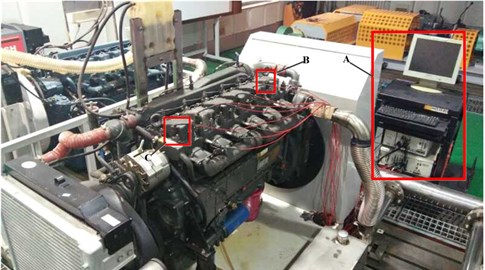#### 3.2. Experimental data processing

The vibration signal of normal operation of diesel engine is displayed in a complete working cycle, as shown in Fig. 2. The vibration signal of the working cycle is decomposed by EWT, the number of spectrum segments is decomposed by $N=$ 5, and the decomposition results are shown in Fig. 3.

From Fig. 3, it can be found that the frequency components of diesel engine vibration signal are more complex, multiple modal components contain noise interference, low frequency noise interference is small, and high frequency component noise interference is obvious. The vibration signal is processed by EWT autocorrelation denoising, as shown in Fig. 4. Compared to Fig. 3 and Fig. 4, it is found that the amplitude of each component is greatly reduced after the noise elimination, and the effect of the noise elimination is remarkable in the whole, but there is still no ideal at the end point.

The denoising and reconstruction signal is shown in Fig. 5. The reconstructed signal eliminates the influence of the periodic noise components, and preserves the periodic components of the original signal, and the denoising effect is obvious. The results show that the EWT autocorrelation denoising method is effective.

Fig. 2. Vibration signal of normal operation of diesel engine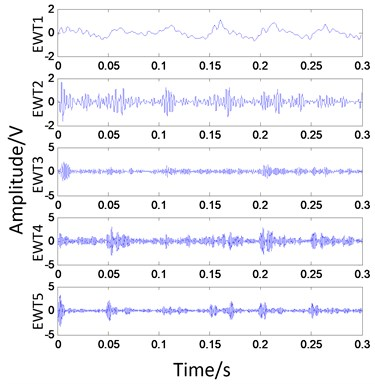Fig. 3. EWT decomposition of vibration signal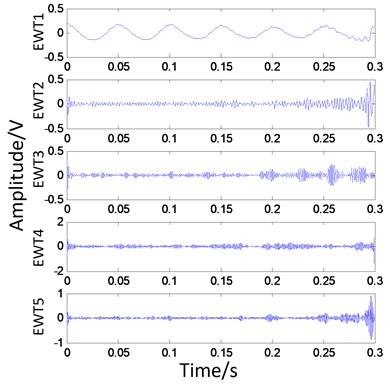Fig. 4. EWT autocorrelation denoising of vibration signal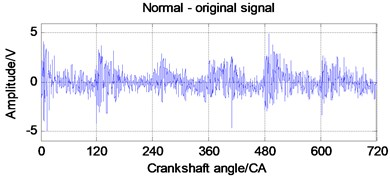Fig. 5. Denoising reconfiguration signal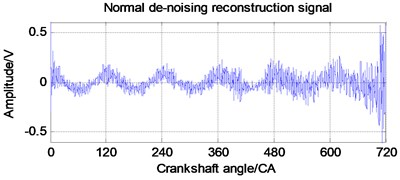#### 4. Conclusions

In this paper, the principle and advantages of empirical wavelet transform (EWT) are expounded. The method of vibration signal denoising based on EWT autocorrelation analysis is proposed. The validity of the method is verified by the actual vibration signal of diesel engine. EWT is essentially a transformation of the Fourier spectrum of the signal. Compared with EMD, it can effectively decompose the intrinsic modal components of the signal, and solve the problem of excessive modal components and false modal components in the EMD decomposition process. The noise elimination method based on EWT autocorrelation can effectively filter the high frequency noise components in the signal and retain the useful information in the signal.

1. Huang Y., Wang K. H., Zhou X. X. Translation invariant wavelet de-noising of CO2 gas shielded arc welding electrical signal. Chinese Journal of Mechanical Engineering, Vol. 54, 2018, p. 95-100. [Publisher]
2. Qian L., Kang M. Gearbox fault diagnosis and its application based on wavelet packet and centroid particle swarm algorithm. Journal of Vibration and Shock, Vol. 35, 2016, p. 191-195. [Search CrossRef]
3. Zhang R., Deng A. D., Si X. D., Liu D. Y., Li J. A new method for acoustic emission signal de-noised and fault diagnosis. Journal of Vibration and Shock, Vol. 37, 2018, p. 75-81. [Search CrossRef]
4. Gilles J. Empirical wavelet transform. IEEE Transactions on Signal Processing, Vol. 61, Issue 16, 2013, p. 3999-4010. [Publisher]
5. Gilles J., Tran G., Osher S. 2D empirical transforms: wavelets, ridgelets and curvelets revisited. SIAM Journal on Imaging Sciences, Vol. 7, Issue 1, 2014, p. 157-186. [Publisher]
6. Kedadouche M., Thomas M., Tahan A. A comparative study between empirical wavelet transforms and empirical mode decomposition methods: application to bearing defect diagnosis. Mechanical Systems and Signal Processing, Vol. 81, 2016, p. 88-107. [Publisher]
7. Hu Yue, Li Fucai, Li Hongguang, Liu Chengliang. An enhanced empirical wavelet transform for noisy and non-stationary signal processing. Digital Signal Processing, Vol. 60, 2017, p. 220-229. [Publisher]
8. Liu Tao, Li Jian, Cai Xiaofeng, Yan Shaoze. A time-frequency analysis algorithm for ultrasonic waves generating from a debonding defect by using empirical wavelet transform. Applied Acoustics, Vol. 131, 2018, p. 16-27. [Publisher]
9. Kedadouche Mourad, Liu Zhaoheng, Vu Viet Hung. A new approach based on OMA-empirical transforms for bearing fault diagnosis. Measurement, Vol. 90, 2016, p. 292-308. [Publisher]
10. Chen Jinglong, Pan Jun, Li Zipeng. Generator bearing fault diagnosis for wind turbine via empirical wavelet transform using measured vibration signals. Renewable Energy, Vol. 89, 2016, p. 80-92. [Publisher]
11. Zhu Y. P., Bao W. J., Tu X. T., Hu Y., Li F. C. Application of enhanced empirical wavelet transform to rolling bearing fault diagnosis. Noise and Vibration Control, Vol. 38, 2018, p. 199-203. [Search CrossRef]
12. Daubechies Ingrid. Ten lectures on wavelets. CBMS-NSF Regional Conference Series in Applied Mathematics, Philadelphia, 1992. [Publisher]
13. Boudraa A. O., Cexus J. C. EMD-based signal filtering. IEEE Transactions on Instrumentation and Measurement, Vol. 56, 2007, p. 2196-2202. [Publisher]